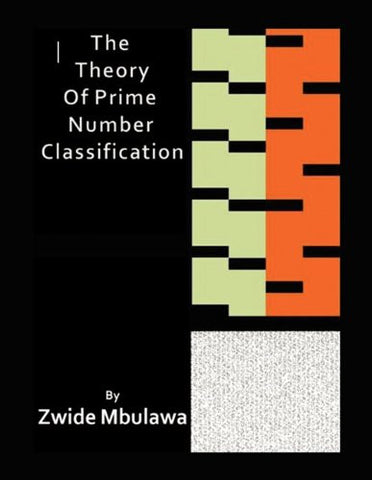Shopping Cart### The Theory of Prime Number Classification

Regular price \$87.76 Sale price

Compare at \$106.88

• ISBN-13: 9781453599822
• Publisher: Xlibris
• Release Date: Feb 25, 2011
• Pages: 204 pages
• Dimensions: 0.53 x 11.02 x 8.5 inches

### Overview

The Theory of Prime Number Classification

This is an expository work of mathematical research into the prime numbers based on pattern methodology and classification techniques. As a comprehensive research into the classification systems for prime numbers, it address the following:

„Why prime numbers are regular yet random.
„What are the building blocks of prime numbers
„What is the framework for prime number generation

This is done by developing the following classification systems:
„The Prime Root Classification. All prime numbers are constituted by roots, which are defined as the building blocks of the prime number.
„The Positional Classification. A two dimensional prime number space is defined that allows certain types of distribution analysis of primes to be made, deriving count functions and establishing the mean property of primes
„The Delta Classification of Primes. This classification creates prime families in terms of gaps. Prime gaps are found to have positive, negative and a steady gap acceleration.
„The Gap Theory Classification. All prime gaps and prime number behavior are based on Gap 2, Gap 4 and Gap 6. This then develops a classification system.

Using the above classification systems, and defining a special function, a theory of prime number generation is then suggested, where this leads to the development of an algebraic sieve for finding prime numbers. The algebraic sieve contains all the relevant information about prime numbers, including how gaps widen, and prime number patterns. Consequently, it is then used to address the problem of finding a proof for the twin prime conjecture.

As an expository work, the book also shares personal experiences and thoughts with regard to the research, and the development of expository mathematics. A program for prime number classification is available at www.zwideprimes.com